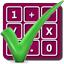#### Arithmetic of Computers

#### from Tenscope Limited

By using this site, you are accepting "session" cookies, as set out in the site's Privacy Policy
Cookies are also used to remember which page of the book you last viewed, so that when you revisit the site you automatically return to the last page you visited.

### Lesson 1

#### Page 10

No.
You are correct. Nowhere does the number 3 appear in the multiplication. The number of factors involved is different from the factors themselves. We can use one factor any number of times.
Now, if we had to write out the multiplication in which 2 is used as a factor nine times, it would be rather cumbersome:
2 × 2 × 2 × 2 × 2 × 2 × 2 × 2 × 2 = 512.
For convenience, there is a shorthand way of writing a phrase such a “the product formed by using 2 as a factor nine times.” The whole phrase may be written simply as “29.” This symbol means
the number formed by using this number as a factor → 29 ← this many times
Thus, 29 means (or equals)
2 × 2 × 2 × 2 × 2 × 2 × 2 × 2 × 2 = 512,
and similarly
23  = 2 × 2 × 2 = 8,  24  = 2 × 2 × 2 × 2 = 16,   etc.
What would the symbol 34mean?